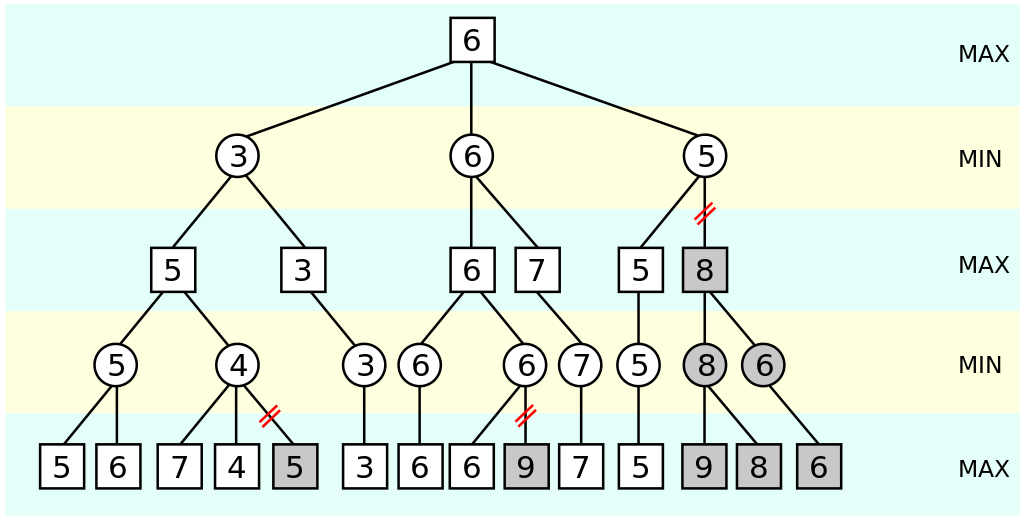## Thinking with Laziness

Tikhon Jelvis (tikhon@jelv.is)

Zoom in/out: Ctrl or Command + +/-

# 1 Perspectives

• modularity
• evaluation vs definition
• control
• lazy structures as control flow
• precision
• arbitrary precision values
• memoization
• laziness = built-in memoization

# 2 Modularity

• separate evaluation from definition
• evaluate at use site
• different use sites—different evaluation
• new way of separating into components
• interleave or modify evaluation at use site

# 3 Preserving Asymptotics

• select n top elements
```select ∷ Ord o ⇒ [o] → [o]
select = take n . sort
```
• does not sort whole list
• like adding `break` into definition of `sort`

# 4 Control Execution

• F18A emulator:
```step ∷ State → State

trace ∷ State → [State]
trace = iterate step
```
• infinite list of states

# 5 Different Uses

• repl: run until end state
• `takeWhile (≠ end) \$ trace start`
• tests:
• `take limit \$ trace start`
• limit based on spec program
• both:
• `take limit . takeWhile (≠ end)`

# 6 α-β pruning• don't evaluate pruned branches

# 7 Control Structures

• lazy data structure ≡ control flow
• list ≡ for loop
• first-class
• manipulate
• pass into functions
• pattern match
• compose
• combine into larger lazy structures

# 8 Examples

• F18a trace
• interpreter loop
• game tree
• recursive move function
• `take n . sort`
• loop
• partially executed sort

# 9 Intermediate Structures

• lazy structures need not fully exist
• garbage collected on the fly
• `fact n = product [1..n]`
• internal list ⇒ for loop
• collected on the fly
• constant memory usage
• common style:
• `fold . unfold`

# 10 Nondeterministic Programming

• lists ≡ loop
• nest list ≡ nested loop
• nondeterministic programming
```do a ← [1..10]
b ← [1..10]
guard (a ≠ b ∧ a + b == 7)
return (a, b)
```

# 11 Map Coloring# 12 Map Coloring# 13 Map Coloring

• `step ∷ Map → State → [Map]`
```solutions ∷ [Map]
solutions = foldM step blank states

-- solution where California is blue
some = find caBlue solution
all = filter caBlue solution
```

# 14 Arbitrary Precision

• lazy structures ⇒ precision on demand
• Conal Elliott:

• modularity!
• vector vs raster

# 15 Exact Real Arithmetic

• lazy list of digits
• continued fractions
• any other series
```N  [1, 4, 1, 5, 9, 2, 6...]
```
• simple implement
• no loss of precision at seams# 17 Memoization

• built-in controlled side-effect
• below level of abstraction
• laziness:
• computes value at most once
• deterministic

# 18 Fibonacci

• classic example
```fibs ∷ [Integer]
fibs = 0 : 1 : zipWith (+) fibs (drop 1 fibs)
```
•# 19 Fibonacci

•••# 20 Intermediate Values

```fib ∷ Integer → Integer
fib n = fibs !! n
where fibs =
0 : 1 : zipWith (+) fibs (drop 1 fibs)
```

-# 21 Intermediate Values

•••# 22 Packages

• Luke Palmer: `data-memocombinators`
• Conal Elliott: `MemoTrie`
• infinite lazy trees

# 23 Dynamic Programming

• array of lazy values
```fib ∷ Integer → Integer
fib 0 = 0
fib 1 = 1
fib n = fibs ! (n - 1) + fibs ! (n - 2)
where
fibs = Array (0, n) [go i | i ← [0..n]]
```

# 24 Dynamic Programming

• array with dependencies as thunks
•• interesting for harder problems!

# 25 Perspectives

• modularity
• evaluation vs definition
• control
• lazy structures as control flow
• precision
• arbitrary precision values
• memoization
• laziness = built-in memoization

# 26 References

• “Why Functional Programming Matters” by John Hughes
• Parallel and Concurrent Programming in Haskell by Simon Marlowe
• “Lazy Algorithms for Exact Real Arithmetic” by Pietro Di Gianantonio and Pier Luca Lanzi
• “Functional Programming and Quadtrees” by F. Warren Burton and John (Yannis) G. Kollias